# What is Maximum Over-Current Protection (MOCP vs MCA vs FLA vs LRA)

Contents

## What Does MOCP Mean in Electrical Terms?

MOCP stands for Maximum Over-Current Protection and is defined as the maximum allowable current rating for the over-current protection devices (such as a fuse or circuit breaker) associated with a piece of electrical equipment (e.g. a motor or air conditioner). MOCP is the maximum permissible rating or size of the circuit breaker that will properly disconnect the circuit or equipment under any anticipated fault condition.

If the protective devices are oversized then it may be unable to operate under fault condition and hence, the wire or equipment may get damaged due to overheating. So, the proper sizing is necessary for protective devices.

The value of MOCP helps us to determine the maximum size of the over-current protection device i.e., fuse and circuit breaker. MOCP can be used to protect the wire and equipment under anticipated fault conditions.

Thus, MOCP or MOP = Maximum Over-Current Protection = Maximum Fuse or Circuit Breaker Rating.

## MCA vs MOCP vs FLA vs LRAs

The information of MCA, MOCP, FLA, and LRA is very important as it is required for safely wiring and protecting the equipment. Let’s discuss it one by one.

### MCA

MCA stands for Minimum Current Ampacity or Minimum Circuit Ampacity is defined as the minimum current ratings for the supply wire or conductor in an electrical circuit. In other words, MCA is the minimum current ratings that the wires or conductors should safely carry under normal operating conditions.

The minimum current ampacity is the amount of the current that the conductor should carry hence it is the current carrying capacity of the conductor or wire.

The value of MCA helps us to determine the minimum wire size to ensure that the wire should not overheat under normal operating conditions.

Thus, MCA = Minimum Current Ampacity = Minimum Wire or Conductor Size

The value of MCA is the 1.25 times the FLA of the motor adding in all other resistive loads i.e., heater load.

MCA = 1.25 * (Motor FLA + Heater Current)

### MOCP

The MOCP is the measured value that is used to determines the maximum size of the overcurrent protection devices such as circuit breaker or fuse that is used to protect the wire and equipment under fault conditions.

The size of the circuit breaker or fuse must be more than the value of minimum current amps (MCA). Hence the value of MOCP is always greater than the value of MCA.

MCA and MOCP are important values that determine the minimum wire/conductor size and maximum fuse/circuit breaker size that is allowed to reduce risks of over-current and hence to reduce fire risk.

The value of MOCP is 2.55 times the FLA of the largest motor adding in all other loads of 1 A or more that could in operation at the same time.

MOCP = (2.25 * FLA of the Largest Motor) + (Other Motor Loads) + (All other resistive electrical load i.e., Heater Load)

### FLA

FLA stands for Full Load Ampere is the amount of continuous current that equipment or machines can draw in running conditions at maximum load. FLA is the full load current at the rated voltage and load that the motor will draw to produce rated output HP.

The value of FLA is more important as it is used to determine the value of MCA and MOCP. Hence, indirectly it is used to determine the size of the conductors, Equipment, overcurrent protection devices such as fuse, MCB, circuit breaker, etc.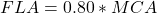and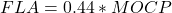### LRA

LRA stands for Locked Rotor Ampere is the amount of the current that the motor can draw in a locked rotor condition. The value of LRA can be nearly equal to the starting current of the motor and about 8 times the full load current.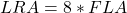The value of LRA is used to calculate the maximum voltage drop at the starting conditions of the motor. If the voltage drop is more than 80 % to 85 % then the motor can refuse to start and it starts vibrating.

## How To Calculate MOCP

The value of MOCP is provided on the nameplate of any equipment or unit by the manufacturer to ensure safe operation. The overcurrent protection device such as the fuse and the circuit breaker are properly sized so that the equipment cannot draw the current more than the rating of the MOCP. We can calculate the value of MOCP based on FLA.

MOCP = (2.25 * FLA of the Largest Motor) + (Other Motor Loads) + (All other resistive electrical load i.e., Heater Load)

The standard current rating of the circuit breaker is 15 A, 20 A, 25 A, 30 A, 35 A ……, 60 A, etc. where 15 A is the minimum current rating of the fuse or circuit breaker permitted by the National Electrical Code in the USA.

There are two types of load in high voltage electrical circuits.

1. Inductive Load i.e., Motor, Compressor, etc.…
2. Resistive Load i.e., Electric Heaters.

### Steps to calculate MOCP

First, find FLA of the Motor or Compressor – It is the Full load Current at the rated voltage and load.

After calculating the value of MOCP, we must select the value of MOCP based on three conditions which are given below.

1. If MOCP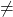Multiple of 5 i.e., if the calculated value of MOCP is not an even multiple of 5 then the value of MOCP is rounded down to the nearest standard fuse or circuit breaker size.
2. If MOCP < MCA i.e., if the calculated value of MOCP is less than the value of MCA then the value of MOCP is taken equal to the MCA and it is rounded up to the nearest standard fuse or circuit breaker size, typically a multiple of 5. Hence the value MOCP is not less than the value of MCA.
3. If MOCP < 15 A i.e., if the calculated value of MOCP is less than 15 A then it is rounded up to 15 A. This 15 A is the minimum current size or rating of the fuse or circuit breaker permitted by code.

Let’s see examples of how to select MOCP value based on the above three conditions.

#### Example 1 : Calculate the MOCP value for 3 – phase, 480 V, 10 KW heater load with motor FLA 4.5 A.

Given Data: Supply Voltage = 3-phase 480 V, Heater load = 10 KW, Motor FLA = 4.5 A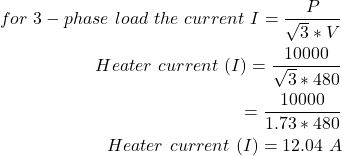Now,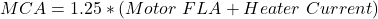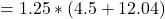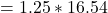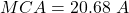and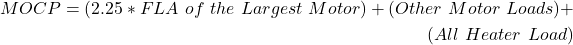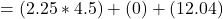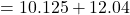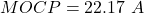Here, the value of MOCP is not an even multiple of 5 hence it is rounded down to the nearest circuit breaker size i.e., 20 A. thus,

MOCP = 20 A (condition 1),

but 20 A is less than the value of MCA hence, the MOCP is taken equal to the value of MCA and it is rounded up to the nearest circuit breaker rating. Thus, MOCP is 25 A for this 3-phase load (Condition 2).

(Note that in USA 277 V is 1 – phase voltage and 480 V is 3 – phase voltage and for India 230 V is 1-phase and 415 V is 3-phase voltage).

#### Example 2: Calculate the MOCP value for 1 – phase, 277 V, 5 KW heater load.

Given Data: Supply Voltage = 1-phase 277 V, Heater load = 5 KW, Motor FLA = 0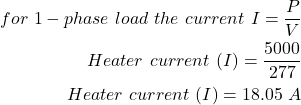Now,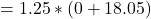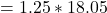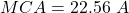and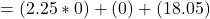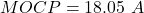Here, MOCP < MCA hence, the value of MOCP is taken equal to the value of MCA and it is rounded up to nearest circuit breaker rating. Thus, MOCP is 25 A for this 1-phase heater load (Condition 2).

#### Example 3: Calculate the MOCP value for 3 – phase, 480 V, 5 KW heater load.

Given Data: Supply Voltage = 3-phase 480 V, Heater load = 5 KW, Motor FLA = 0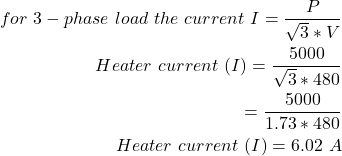Now,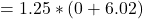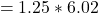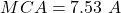and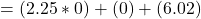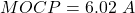Here, MOCP < 15 A hence, the value of MOCP is rounded up to 15 A which is the minimum current rating of the circuit breaker (Condition 3).

## How To Calculate MCA

The value of MCA is provided on the nameplate of the any equipment or unit by the manufacturer to ensure safe operation. We can calculate the value of MCA by the calculating the value of FLA.

To calculate the value of MCA, we must calculate the current rating of all the other equipment’s i.e., fan, motor, compressors, etc….

MCA = 1.25 * (Motor FLA + Heater Current)

Let’s see one example of how to calculate the value of MCA.

### Example: Calculate the MOCP value for 3 – phase, 480 V, 12 KW heater load with motor FLA 5 A.

Given Data: Supply Voltage = 3-phase 480 V, Heater load = 12 KW, Motor FLA = 5 A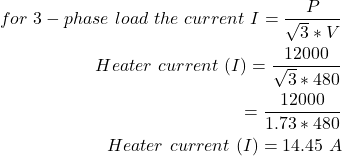Now,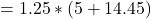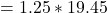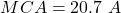Thus, the value of MCA is 20.7 A.

As discussed above that the value of MOCP and MCA are provided on the nameplate of equipment. It is shown in the below nameplate.

As shown in the nameplate, the Maximum size or rating of the fuse or circuit breaker is 20 A it means that the value of MOCP is 20 A. Hence, we can select the overcurrent protective devices as per the above MOCP rating.

Similarly, the Minimum circuit ampere is 12.2 A it means that the value of MCA is 12.2 A. Thus, we can select the minimum size wire as per the MCA rating.

The value of LRA and FLA of the fan motor is also provided.

Want To Learn Faster? 🎓
Get electrical articles delivered to your inbox every week.
No credit card required—it’s 100% free.